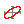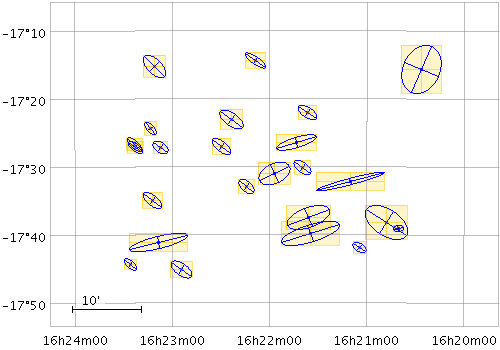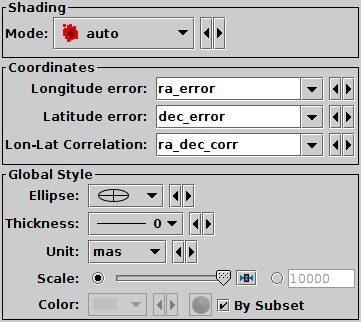Next Previous Up Contents
Next: Polygon Form
Up: Plot Forms
Previous: XYCorr Form

#### A.4.5.10 SkyCorr Form

The SkyCorr () form plots an error ellipse (or rectangle or other similar figure) on the sky defined by errors in the longitude and latitude directions, and a correlation between the two errors.

The error in longitude is considered to be premultiplied by the cosine of the latitude, i.e. both errors correspond to angular distances along a great circle.

The supplied correlation is a dimensionless value in the range -1..+1 and is equal to the covariance divided by the product of the lon and lat errors. The covariance matrix is thus:

```   [  lonerr*lonerr       lonerr*laterr*corr  ]
[  lonerr*laterr*corr  laterr*laterr       ]
```

This plot type is suitable for use with the `ra_error`, `dec_error` and `ra_dec_corr` columns in the Gaia source catalogue. Note however that Gaia positional errors are generally quoted in milli-arseconds (mas), while this plotter requires lon/lat errors in degrees, so to plot true error ellipses it is necessary to divide the Gaia error values by 3.6e6. In most plots, Gaia position errors are much too tiny to see!Example SkyCorr plotSkyCorr form configuration panel

The configuration options are:

See Appendix A.4.6.
Longitude error
Error in longitude in degrees, multiplied by the cosine of the latitude.
Latitude error
Error in latitude in degrees.
Lon-Lat correlation
Correlation between the errors in longitude and latitude. This is a dimensionless quantity in the range -1..+1, and is equivalent to the covariance divided by the product of the Longitude and Latitude error values themselves. It corresponds to the `ra_dec_corr` value supplied in the Gaia source catalogue.
Ellipse
Ellipse graphical representation, selected from a range of options (includes also rectangles, crosses etc).
Thickness
Controls the line thickness used when drawing ellipses. Zero, the default value, means a 1-pixel-wide line is used. Larger values make drawn lines thicker, but note changing this value will not affect all shapes, for instance filled polygons contain no line drawings.
Unit
Gives the units in which the Longitude and Latitude errors are supplied. Options are degrees, arcseconds etc. If the special value scaled is given then a non-physical scaling is applied to the input values to make the the largest markers appear at a reasonable size (a few tens of pixels) in the plot. Note that the actual plotted size of the markers can also be scaled using the scale option; these two work together to determine the actual plotted sizes.
Scale
Scales the size of plotted ellipses. The default value is 1, smaller or larger values multiply the visible sizes accordingly. The main purpose of this option is to tweak the visible sizes of the plotted markers for better visibility. The unit option is more convenient to account for the units in which the angular extent coordinates are supplied. If the markers are supposed to be plotted with their absolute angular extents visible, this option should be set to its default value of 1.

Next Previous Up Contents
Next: Polygon Form
Up: Plot Forms
Previous: XYCorr Form

TOPCAT - Tool for OPerations on Catalogues And Tables